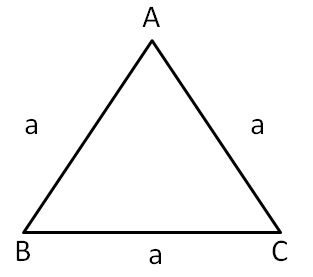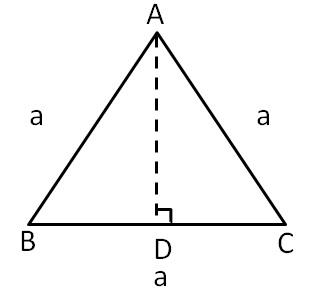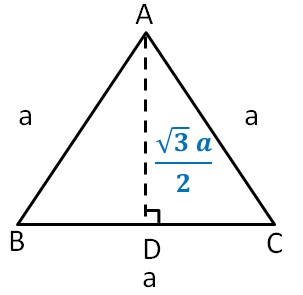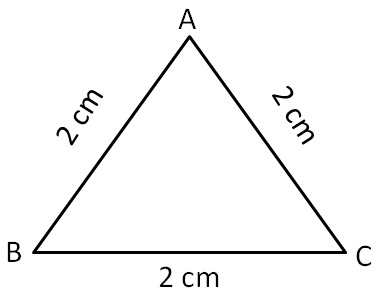Area of triangle

Chapter 9 Class 7 Perimeter and Area
Concept wise

Let there be an equilateral ABCWe need to find its area

We know that,

Area ∆ABC = 1/2 × Base × Height

Finding base & height of equilateral triangle ABC

Height is perpendicular from the vertex to the base.

Let us draw perpendicular from point ASo,

Base = BC = a

So, we need to find height AD

In equilateral triangle,

altitude is also the median

So, point D is also the mid-point of BC

Therefore,

BD = DC = a/2

By Pythagoras theorem

AC 2 = AD 2 + DC 2

a 2 = AD 2 + (a/2) 2a 2 = AD2 + a 2/4

AD^2 + a2/4 = a 2

AD^2 = (4a 2   - a2   )/4

Now,

= √3/2 a

Base = BC

= a

Area of ∆ABC = 1/2 × Base × Height

= 1/2 × a × √3/2 a

= √3/4 a^2

∴ Area of equilateral triangle = √3/4 a 2

Find area of the following equilateral triangle whose sides are 2 cmSide = a = 2 cm

Area of equilateral ∆ABC = √3/4 a 2

= √3/4 (2) 2

= √3/4 × 4

= √3 cm 2

∴ Area of equilateral triangle ∆ABC is √3 cm 2

Learn in your speed, with individual attention - Teachoo Maths 1-on-1 Class